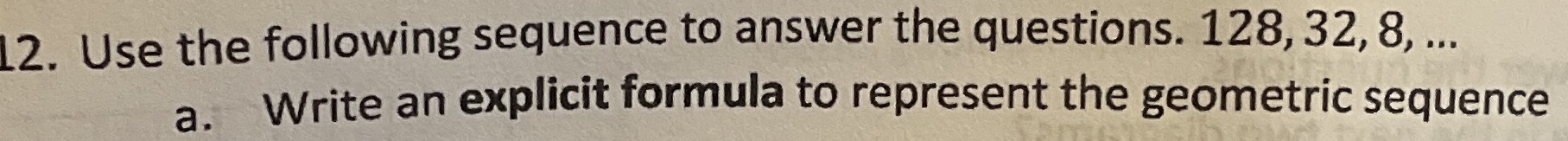### ¿Todavía tienes preguntas de matemáticas?

Pregunte a nuestros tutores expertos
Algebra
Pregunta12. Use the following sequence to answer the questions. $$128,32,8 , \ldots$$

a. Write an explicit formula to represent the geometric sequence

$$a_n= 128\times(\frac{1}{4} )^{n- 1}$$